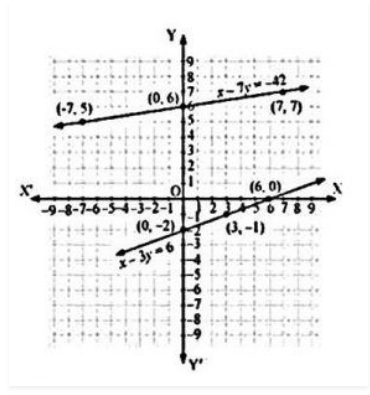# Aftab tells his daughter, “Seven years ago, I was seven times as old as you were then. Also, three years from now, I shall be three times as old as you will be.” (Isn’t this interesting?) Represent this situation algebraically and graphically.

Let the current age of Aftab be ‘x’.

And, the current age of his daughter be ‘y’.

Now, we can write, seven years ago,

Age of Aftab = x-7

Age of his daughter = y-7

According to the given condition

x−7 = 7(y−7)

⇒x−7 = 7y−49

⇒x−7y = −42 ………………………(i)

Also, three years from now or after three years,

Age of Aftab will become = x+3.

Age of his daughter will become = y+3

According to the situation given,

x+3 = 3(y+3)

⇒x+3 = 3y+9

⇒x−3y = 6 …..…………………(ii)

Subtracting equation (i) from equation (ii) we get

(x−3y)−(x−7y) = 6−(−42)

⇒−3y+7y = 6+42

⇒4y = 48

⇒y = 12

The algebraic equation can be solved as

x−7y = −42

x−3y = 6

For, x−7y = −42 or x = −42+7y

The solution table is

 x -7 0 7 y 5 6 7

For, x−3y = 6 or x = 6+3y

The solution table is

 x 6 4 0 y 0 -1 -2

The graphical representation is(40)(11)